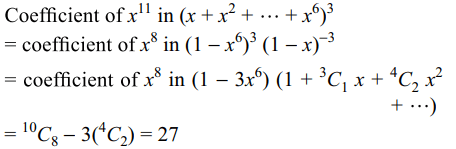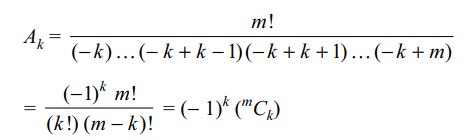## Permutations and Combinations Questions and Answers Part-8

1. The number of ways in which a committee of 3 women and 4 men be chosen from 8 women and 7 men if Ms. X refuses to serve on the committee if Mr. Y is a member of the committee is
a) 420
b) 840
c) 1540
d) 720

Explanation:2. The product of first n odd natural numbers equals
a) $\left(^{2n}C_{n}\right)\left(^{n}P_{n}\right)$
b) $\left(\frac{1}{2}\right)^{n}\left(^{2n}C_{n}\right)\left(^{n}P_{n}\right)$
c) $\left(\frac{1}{4}\right)\left(^{2n}C_{n}\right)\left(^{2n}P_{n}\right)$
d) $\left(\frac{1}{n!}\right)\left(^{2n}C_{n}\right)$

Explanation:3. The number of integral solutions of x + y + z = 0 with x ≥ – 5, y ≥ – 5, z ≥ – 5 is
a) 134
b) 136
c) 138
d) 140

Explanation:4. A library has n different books and has p copies of each of the book. The number of ways of selecting one or more books from the library is
a) $n^{p}$
b) $p^{n}$
c) $\left(n+1\right)^{p}-1$
d) $\left(p+1\right)^{n}-1$

Explanation: For each book we have (p + 1) choices.

5. The number of ways in which we can get a score of 11 by throwing three dice is
a) 18
b) 27
c) 45
d) 56

Explanation:6. 2m white counters and 2n red counters are arranged in a straight line with (m + n) counters on each side of a central mark. The number of ways of arranging the counters, so that the arrangements are symmetrical with respect to the central mark, is
a) $^{m+n}C_{m}$
b) $^{2m+2n}C_{2m}$
c) $\frac{1}{2}\frac{\left(m+n\right)!}{m!n!}$
d) mn

Explanation: Arrange m white and n red counters on one side of the central mark.

7. Let x = (2n + 1) (2n + 3) …. (4n – 3) (4n – 1) and $y=\left(\frac{1}{2}\right)^{n}\frac{\left(4n\right)!n!}{\left(2n\right)!\left(2n\right)!}$       then $x-y+2^{n}$    is equal to
a) 0
b) $\left(2n\right)!/2^{n}$
c) $^{2n}C_{n}$
d) $2^{n}$

Explanation:8. There are 3 set of parallel lines containing respectively p lines, q lines and r lines respectively.The greatest number of parallelograms that can be formed by the system
a) pqr + (p – 1) (q – 1) (r – 1)
b) $\frac{1}{4}\left\{pqr+\left(p-1\right)\left( q-1 \right)\left(r-1\right)\right\}$
c) $\frac{1}{4}pqr\left(p+1\right)\left( q+1 \right)\left(r+1\right)$
d) $\left(^{p}C_{2}\right)\left( ^{q}C_{2} \right)+\left(^{q}C_{2}\right)\left(^{r}C_{2}\right)+\left(^{r}C_{2}\right)\left(^{p}C_{2}\right)$

Explanation:9.The number of six-digit numbers which have sum of their digits as an odd integer, is
a) 45000
b) 450000
c) 97000
d) 970000

10. In the identity $\frac{m!}{x \left(x+1\right) \left(x+2\right) ....\left(x+m\right)}=\sum_{i=0}^{m}\frac{A_{i}}{x+i}$         , then value of $A_{k}$ is
a) $^{m}C_{k}$
b) $^{m}C_{k+1}$
c) $\left(-1\right)^{k}\left(^{m}C_{k}\right)$
d) $^{m}C_{k-1}$